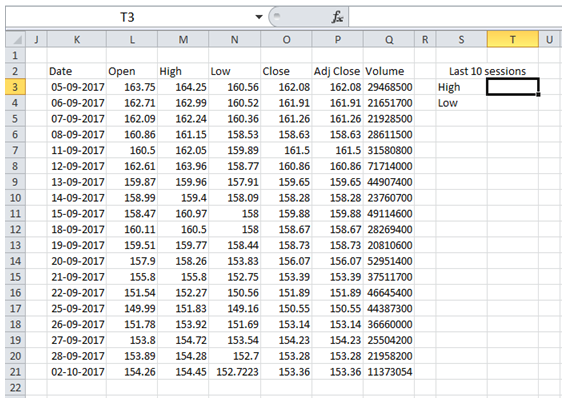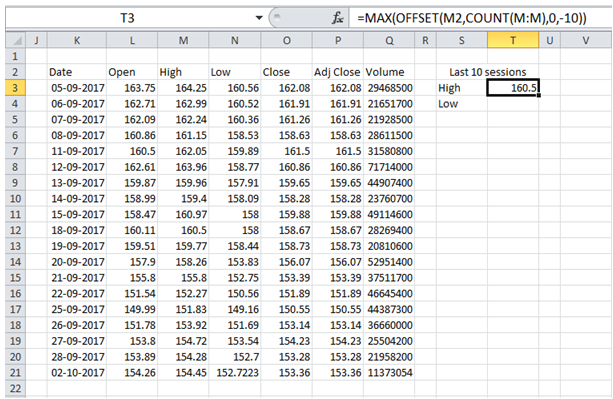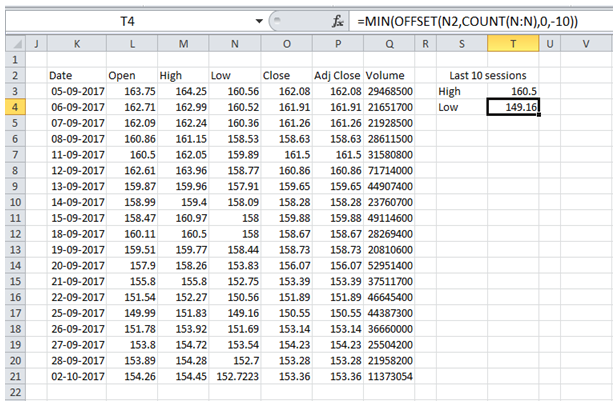## Get last 10 entries in a table in Excel

Table below is the raw data that I capture. I update one row for each trading day. I want to set up the Worksheet to automatically calculate the HIGH and LOW values for the last 10 sessions.To do it in Excel, here is the answer:

a) High value in last 10 trading sessions corresponds to Max value among last 10 values in Col "High" (Col M).

Enter formula =MAX(OFFSET(M2,COUNT(M:M),0,-10)) to get High value in last 10 trading sessions as shown below.

The first argument of OFFSET function refers to the Title in column as reference.

COUNT(M:M) counts numeric values in Column M (Title "High" is excluded since it is a text) and returns reference to the last cell with numeric value in Column M (M21)*** .

The fourth argument -10 extends the range referred by OFFSET by 10 rows above the last cell M21 to create the range M12:M21.

Max formula computes the maximum value in range M12:M21

***assuming no blank rows or rows with text in column M.b) Low value in last 10 trading sessions corresponds to Min value among last 10 values in Col "Low" (Col N).

Enter formula =MIN(OFFSET(N2,COUNT(N:N),0,-10)) to get Low value in last 10 trading sessions as shown below.

The first argument of OFFSET function refers to the Title in column as reference.

COUNT(N:N) counts numeric values in Column N (Title "Low" is excluded since it is a text) and returns reference to the last cell with numeric value in Column N (N21)*** .

The fourth argument -10 extends the range referred by OFFSET by 10 rows above the last cell N21 to create the range N12:N21.

Min formula computes the maximum value in range N12:N21

***assuming no blank rows or rows with text in column N.